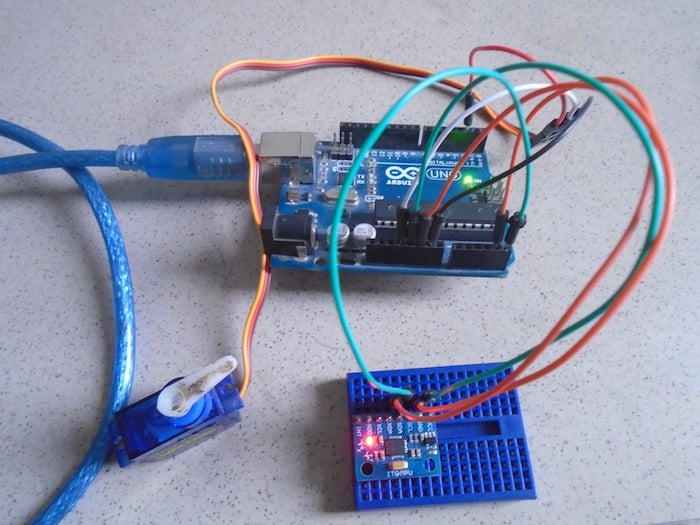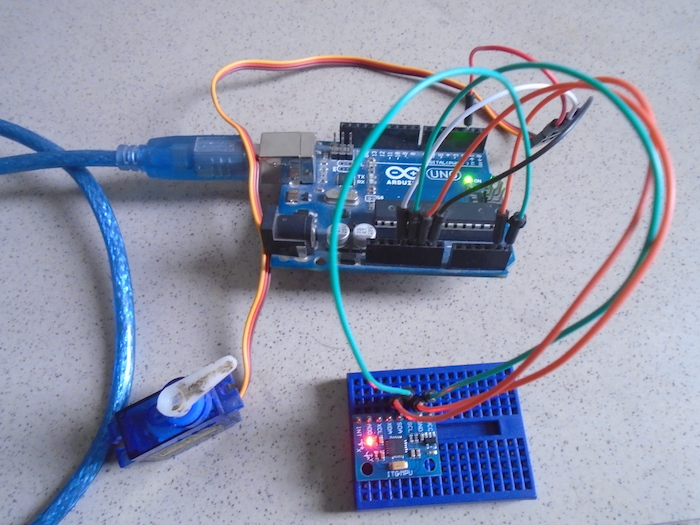Arduino

# How to Control a Servo With an Arduino and MPU6050March 23, 2018 by A .
ShareLearn how to control a servo motor using an MPU6050 sensor connected to an Arduino.

In this project, we are going to control a servo motor using the MPU6050 (accelerometer and gyro) sensor. We will connect the servo motor and the MPU6050 sensor with the Arduino in such a way that whenever we move the MPU6050, the servo motor will move according to it.

### How Does It Work?

The structure of the accelerometer is a mass attached to a spring which has fixed outer plates and moves along one direction. The capacitance between the plates will change whenever acceleration is applied. This change in capacitance will be measured and will correspond to an acceleration value.

Upon moving the sensor in the upward or downward direction, it gives us values from -17000 to 17000. We will map these from 0 to 180 to move the servo motor. Now when we move the sensor in the upward direction, the output from the sensor will be 180. When we move the move the sensor in the downward direction, then the output of the sensor will be 0.### Circuit Diagram

First of all, connect the MPU 6050 sensor to the Arduino. The connections of the MPU6050 and Arduino are as follows:

• Connect VCC on the MPU6050 to the 5V pin on the Arduino.
• Connect GND on the MPU6050 to the GND on the Arduino.
• Connect SCL on the MPU6050 to A5 on the Arduino.
• Connect SDA on the MPU6050 to A4 on the Arduino.

The A4 and A5 pins on the Arduino are for SPI communication. Thatâ€™s why we have connected SCL and SDA on the MPU6050 to A5 and A4 on the Arduino. After making the connections for the MPU6050 and the Arduino, connect the servo motor to the Arduino. The connections for the servo motor with the Arduino are as follows:

• Connect the servo motor's yellow wire to pin 2 on the Arduino.
• Connect the servo motor's red wire to the 5V pin on the Arduino.
• Connect the servo motor's black wire to GND on the Arduino.### Arduino Code

``````#include <Wire.h>

#include <MPU6050.h>

#include <Servo.h>

Servo sg90;

int servo_pin = 2;

MPU6050 sensor ;

int16_t ax, ay, az ;

int16_t gx, gy, gz ;

void setup ( )

{

sg90.attach ( servo_pin );

Wire.begin ( );

Serial.begin  (9600);

Serial.println  ( "Initializing the sensor" );

sensor.initialize ( );

Serial.println (sensor.testConnection ( ) ? "Successfully Connected" : "Connection failed");

delay (1000);

Serial.println ( "Taking Values from the sensor" );

delay (1000);

}

void loop ( )

{

sensor.getMotion6 (&ax, &ay, &az, &gx, &gy, &gz);

ax = map (ax, -17000, 17000, 0, 180) ;

Serial.println (ax);

sg90.write (ax);

delay (200);

}``````

### Code Explanation

First of all, we included the library for the MPU6050 sensor and the servo motor. The wire library is for the SPI communication between the Arduino and the MPU6050 sensor.

``````#include <Wire.h>

#include <MPU6050.h>

#include <Servo.h>``````

Then we created a variable of name sg90 and of type Servo. This variable will help us in using the functions of the servo library. Then we initialized pin 2 for the servo motor.

``````Servo sg90;

int servo_pin = 2;``````

Then we created a variable of the sensor's name and type: MPU6050. This variable will help us with using the functions of the library. Then we initialized some variables which we will use to measure the output of the MPU6050 sensor.

``````MPU6050 sensor ;

int16_t ax, ay, az ;

int16_t gx, gy, gz ;``````

In the setup function, we attached the servo o the pin 2 on the Arduino. This means that Arduino will give input to the Servo motor through that pin. Then we started the wire communication and after that, we started the serial communication at 9600 baud rate.

``````sg90.attach ( servo_pin );

Wire.begin ( );

Serial.begin  (9600);``````

Then we checked if the MPU6050 sensor is working fine or not. If it is working fine, then "Taking Values from the sensor" will be printed on the screen. If it is not connected, then â€œConnection failed" will be printed on the screen.

``````Serial.println (sensor.testConnection ( ) ? "Successfully Connected" : "Connection failed");

delay (1000);

Serial.println ( "Taking Values from the sensor" );

delay (1000);``````

In the loop function, we took the output from the MPU6050 sensor and mapped this output to the value through which we can control servo. Then we give this value to the servo motor to move it.

``````sensor.getMotion6 (&ax, &ay, &az, &gx, &gy, &gz);

ax = map (ax, -17000, 17000, 0, 180) ;

Serial.println (ax);

sg90.write (ax);

delay (200);``````

### AuthorA .

For custom projects, hire me at https://www.freelancer.pk/u/Muhammadaqibdutt